### Balanced Repeated Replication (BRR)

If you specify the VARMETHOD=BRR option, then PROC SURVEYFREQ uses balanced repeated replication (BRR) for variance estimation. The BRR variance estimation method requires a stratified sample design with two PSUs in each stratum. You can provide replicate weights for BRR variance estimation by using a REPWEIGHTS statement, or the procedure can construct replicate weights for the analysis. PROC SURVEYFREQ estimates the parameter of interest (a proportion, total, odds ratio, or other statistic) from each replicate, and then uses the variability among replicate estimates to estimate the overall variance of the parameter estimate. See Wolter (1985) and Lohr (2009) for more information about BRR variance estimation.

If you do not provide replicate weights with a REPWEIGHTS statement, PROC SURVEYFREQ constructs replicates based on the stratified design with two PSUs in each stratum. This section describes replicate construction by the traditional BRR method. If you specify the FAY method-option for VARMETHOD=BRR, the procedure uses Fay’s modified BRR method, which is described in the section Fay’s BRR Method.

With the traditional BRR method, each replicate is obtained by deleting one PSU per stratum according to the corresponding Hadamard matrix of dimension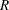, whereis the number of replicates. The number of replicates equals the smallest multiple of 4 that is greater than the number of strata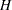. Alternatively, you can specify the number of replicates with the REPS= method-option. If a Hadamard matrix cannot be constructed for the REPS= value that you specify, the value is increased until a Hadamard matrix of that dimension can be constructed. Therefore, it is possible for the actual number of replicates used to be larger than the REPS= value that you specify.

You can provide a Hadamard matrix for BRR replicate construction by using the HADAMARD= method-option. Otherwise, PROC SURVEYFREQ generates an appropriate Hadamard matrix. See the section Hadamard Matrix for more information. You can display the Hadamard matrix by specifying the PRINTH method-option.

PROC SURVEYFREQ constructs replicates by using the firstcolumns of the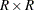Hadamard matrix, wheredenotes the number of strata. Theth replicate (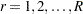) is drawn from the full sample according to theth row of the Hadamard matrix as follows:

• If the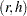th element of the Hadamard matrix equals 1, then the first PSU of stratumis included in theth replicate, and the second PSU of stratumis excluded.

• If theth element of the Hadamard matrix equals –1, then the second PSU of stratumis included in theth replicate, and the first PSU of stratumis excluded.

For the PSUs included in replicate, the original weights are doubled to form the replicateweights. For the PSUs not included in replicate, the replicateweights equal zero. You can use the OUTWEIGHTS=SAS-data-set method-option to store the replicate weights in a SAS data set. See the section Replicate Weights Output Data Set for details about the contents of the OUTWEIGHTS= data set. You can provide these replicate weights to the procedure for subsequent analyses by using a REPWEIGHTS statement.

Let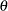denote the population parameter to be estimated—for example, a proportion, total, odds ratio, or other statistic. Let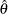denote the estimate offrom the full sample, and let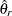denote the estimate from theth BRR replicate, which is computed by using the replicate weights. The BRR variance estimate foris computed as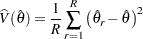whereis the total number of replicates.

If a parameter cannot be estimated from some replicate(s), then the variance estimate is computed by using those replicates from which the parameter can be estimated. For example, suppose the parameter is a column proportion—the proportion of columnfor table cell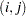. If a replicatecontains no observations in column, then the columnproportion is not estimable from replicate. In this case, the BRR variance estimate is computed as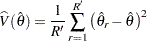where the summation is over the replicates where the parameteris estimable, and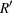is the number of those replicates.

#### Fay’s BRR Method

If you specify the FAY method-option for VARMETHOD=BRR, then PROC SURVEYFREQ uses Fay’s BRR method, which is a modification of the traditional BRR variance estimation method. As for traditional BRR, Fay’s method requires a stratified sample design with two PSUs in each stratum. You can provide replicate weights by using a REPWEIGHTS statement, or the procedure can construct replicate weights for the analysis. PROC SURVEYFREQ estimates the parameter of interest (a proportion, total, odds ratio, or other statistic) from each replicate, and then uses the variability among replicate estimates to estimate the overall variance of the parameter estimate.

If you do not provide replicate weights with a REPWEIGHTS statement, PROC SURVEYFREQ constructs replicates based on the stratified design with two PSUs in each stratum. As for traditional BRR, the number of replicatesequals the smallest multiple of 4 that is greater than the number of strata, or you can specify the number of replicates with the REPS= method-option. You can provide a Hadamard matrix for replicate construction by using the HADAMARD= method-option, or PROC SURVEYFREQ generates an appropriate Hadamard matrix.

The traditional BRR method constructs half-sample replicates by deleting one PSU per stratum according to the Hadamard matrix and doubling the original weights to form replicate weights. Fay’s BRR method adjusts the original weights by a coefficient, where 0
1. You can specify the value ofwith the FAY= method-option. If you do not specify the value of, PROC SURVEYFREQ uses= 0.5 by default. See Judkins (1990) and Rao and Shao (1999) for information about the value of the Fay coefficient. When= 0, Fay’s method becomes the traditional BRR method. See Dippo, Fay, and Morganstein (1984), Fay (1989), and Judkins (1990) for more information.

PROC SURVEYFREQ constructs Fay BRR replicates by using the firstcolumns of theHadamard matrix, wheredenotes the number of strata. Theth replicate () is drawn from the full sample according to theth row of the Hadamard matrix as follows:

• If theth element of the Hadamard matrix equals 1, the sampling weight of the first PSU in stratumis multiplied by, and the sampling weight of the second PSU is multiplied by (2 –) to form theth replicate weights.

• If theth element of the Hadamard matrix equals –1, then the sampling weight of the second PSU in stratumis multiplied by, and the sampling weight of the first PSU is multiplied by (2 –) to form theth replicate weights.

You can use the OUTWEIGHTS= method-option to store the replicate weights in a SAS data set. See the section Replicate Weights Output Data Set for details about the contents of the OUTWEIGHTS= data set. You can provide these replicate weights to the procedure for subsequent analyses by using a REPWEIGHTS statement.

Letdenote the population parameter to be estimated—for example, a proportion, total, odds ratio, or other statistic. Letdenote the estimate offrom the full sample, and letdenote the estimate from theth BRR replicate, which is computed by using the replicate weights. The Fay BRR variance estimate foris computed as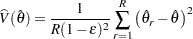whereis the total number of replicates andis the Fay coefficient.

If you request Fay’s BRR method and also include a REPWEIGHTS statement, PROC SURVEYFREQ uses the replicate weights that you provide and includes the Fay coefficientin the denominator of the variance estimate in the preceding expression.

If a parameter cannot be estimated from some replicate(s), then the variance estimate is computed by using those replicates from which the parameter can be estimated. For example, suppose the parameter is a column proportion—the proportion of columnfor table cell. If a replicatecontains no observations in column, then the columnproportion is not estimable from replicate. In this case, the BRR variance estimate is computed as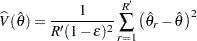where the summation is over the replicates where the parameteris estimable, andis the number of those replicates.

A Hadamard matrix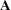of dimensionis a square matrix that has all elements equal to 1 or –1. A Hadamard matrix must satisfy the requirement that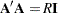, whereis an identity matrix. The dimension of a Hadamard matrix must equal 1, 2, or a multiple of 4.
For example, the following matrix is a Hadamard matrix of dimension= 8:
For BRR replicate construction, the dimension of the Hadamard matrix must be at least, wheredenotes the number of first-stage strata in your design. If a Hadamard matrix of a given dimension exists, it is not necessarily unique. Therefore, if you want to use a specific Hadamard matrix, you must provide the matrix as a SAS data set in the HADAMARD=SAS-data-set method-option. You must ensure that the matrix that you provide is actually a Hadamard matrix; PROC SURVEYFREQ does not check the validity of your Hadamard matrix.